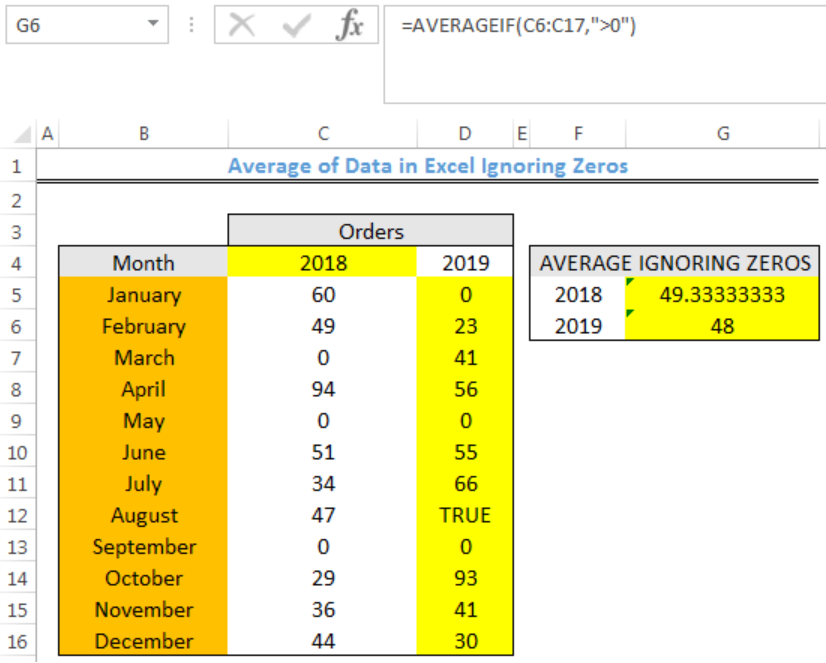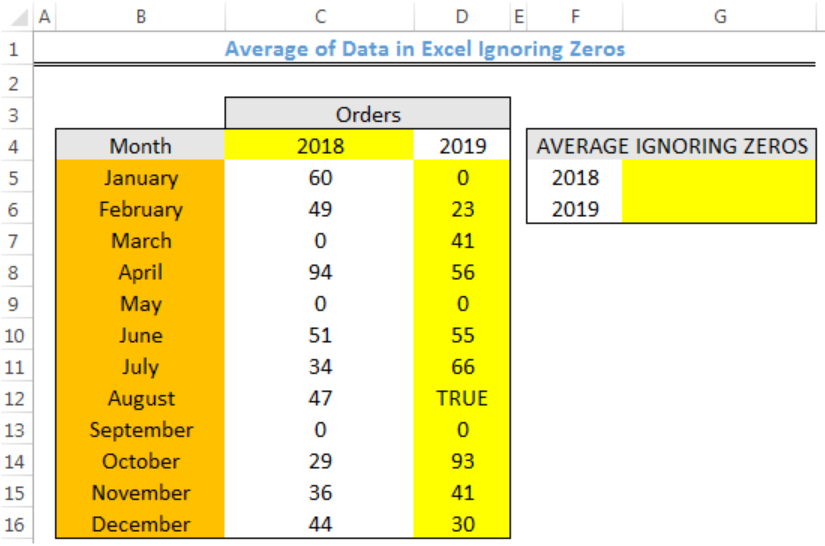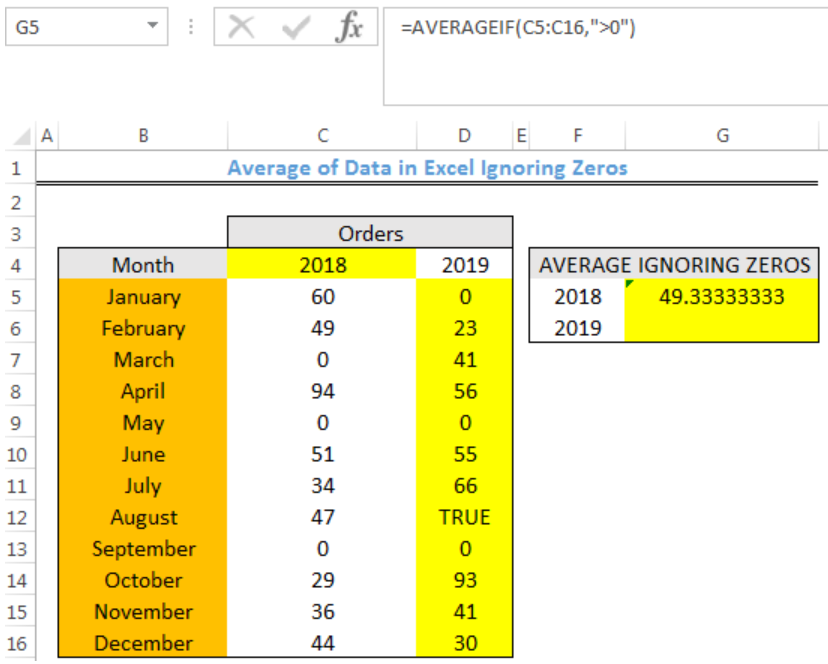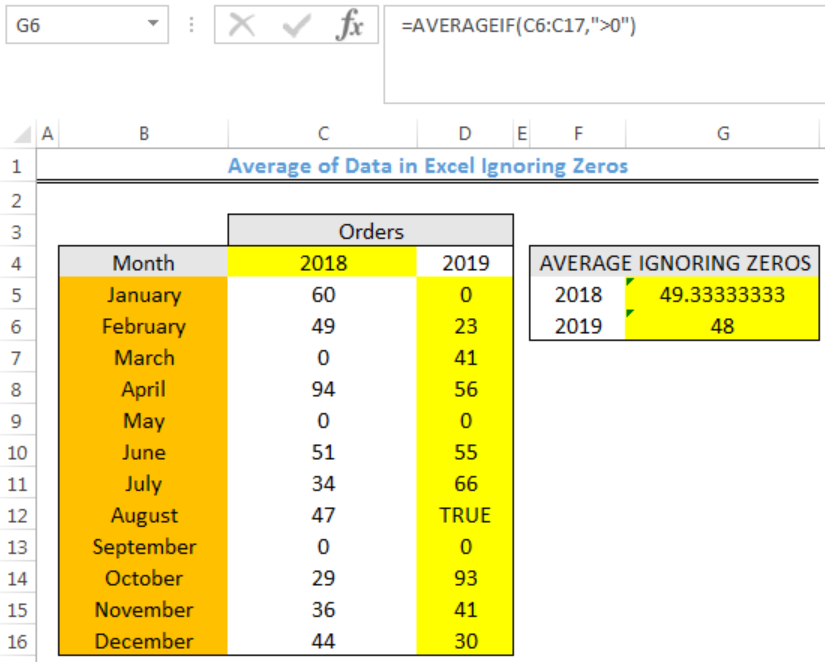Get instant live expert help with Excel or Google Sheets“My Excelchat expert helped me in less than 20 minutes, saving me what would have been 5 hours of work!”

#### Post your problem and you’ll get expert help in seconds.

Your message must be at least 40 characters
Our professional experts are available now. Your privacy is guaranteed.

# Here is How to Find the Average of Data in Excel Ignoring Zeros

We can use the AVERAGEIF function to calculate the average value for a set of values in excel if not 0. Logical operators like >, <, <>, = and wildcards (*,?) for partial matching are supported by the criteria of AVERAGEIF. The steps below will walk through the process.Figure 1- Final result of Average if not 0

## Syntax

`=AVERAGEIF(range,criteria,[average_range])`

• Range: This could be one or more cells, a named range, arrays, or references.
• Criteria: This could be a number, an expression, cell reference, or text.
• Average_range [optional]: These are the cells to get the average. If we omit it, range is used.

## Formula

`=AVERAGEIF(C5:C16,">0")`

## Setting up the Data

We will calculate the average number of orders for the year 2018 and 2019 while ignoring zeros in figure 2.

• The months will be entered into Column B
• Column C and D contain the Orders for 2018 and 2019 respectively
• The average number of orders while ignoring zeros for each year will be returned in Column GFigure 2 – Setting up the Data

## Excel Average if not zero for 2018 and 2019

• We will click on Cell G5
• We will insert the formula below into Cell G5
`=AVERAGEIF(C5:C16,">0")`
• We will press the enter keyFigure 3- Excel Average if not zero for 2018

• We will click on Cell G5 again
• We will double click on the fill handle tool which is the small plus sign you see at the bottom right of Cell G5. Select and drag down to copy the formula to Cell G6.Figure 4- Result of Excel Average if not zero for 2018 and 2019

## Explanation

The AVERAGEIF function checks the range (C5:C16 and D5:D16) for values that are greater than zero. If there are such values, then the average of only those values are returned as the result.

## Note

• Any empty cell and cell with logical values within the range are ignored by the AVERAGEIF Function
• The error value #DIV/0! will be returned if there aren’t cells in the range that meet the criteria

## Instant Connection to an Expert through our Excelchat Service

Most of the time, the problem you will need to solve will be more complex than a simple application of a formula or function. If you want to save hours of research and frustration, try our live Excelchat service! Our Excel Experts are available 24/7 to answer any Excel question you may have. We guarantee a connection within 30 seconds and a customized solution within 20 minutes.

Are you still looking for help with the Average function? View our comprehensive round-up of Average function tutorials here.

Solution examplesI want to calculate an average of some values in a pivot table, however I cannot do that as some rows contain blanks.
Solved by I. C. in 58 minsI need help calculating average time elapsed. It is in dd:hh:mm:ss text format and so I can't calculate using =AVERAGEIF
Solved by O. E. in 11 minsHow do I calculate the average for a group of data that is referenced to multiple cells? I.e. If I wanted to know the average salary of 10 employees with the same salary grade but in different departments.
Solved by O. J. in 40 minsI contacted you yesterdaya bout how to use the =AVERAGE function, and for real numbers it works fine, but I tried to get the expert to explain to me how to use the =AVERAGE function when the cells have formulas in them that generate '0' value, so the AVERAGE function would ignore these cells in the averaging method, but got no answer. So, I need to know how use the =AVERAGE function so that when there are formulas in the selected cells to average, that EXCEL would ignore those cells like they have real numbers in them, which seems to work fine. Please advise...
Solved by C. J. in 30 minsI just need to edit this formula, {=IFERROR(AVERAGEIFS(B6:D6,F6:H6,"FALSE"),AVERAGEIF(B6:D6,"<>0"))}, to return "-" or "0" if there is nothing in column D. I can share the entire worksheet if need be.
Solved by V. A. in 47 mins